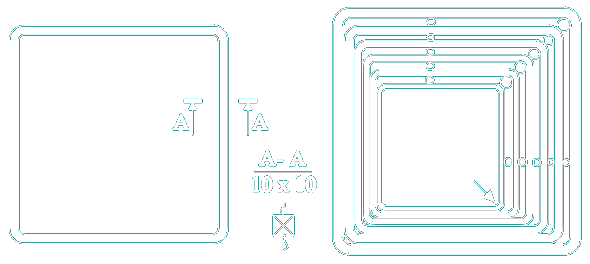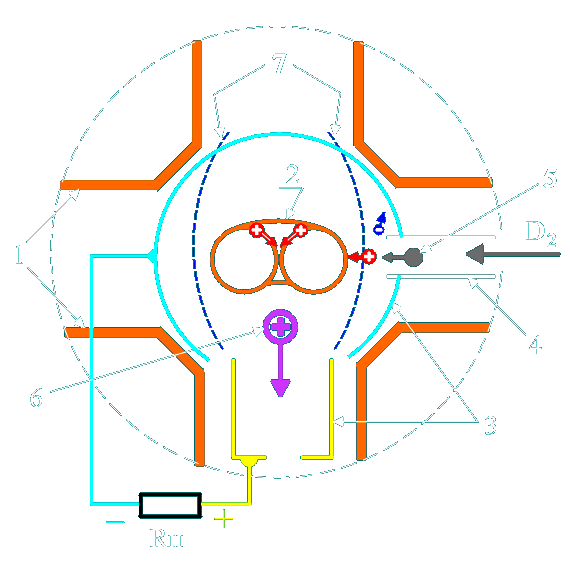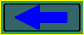﻿ Thermonuclear Reactor of Eugene Grigor'ev (TREG) G21B1/00

Thermonuclear Reactor of  Eugene Grigor'ev (TREG) G21B1/00

The possible principle of construction of an industrial thermonuclear reactor is shown.

The urgency of a problem of using an energy of controllable thermonuclear synthesis is known. This problem till now is not solved because of impossibility of a long-time plasma confinement with temperature more than 100 000 000 degrees. The lack of a magnetic field (MF) with the closed configuration and minimum of magnetic intensity hinders this. That reduces to plasma instability Such a MF can be created by the system from two coaxial toruses, as shown in "Equations of Maxwell".
One of possible construction on Fig. 1 is shown.Fig. 1

On Fig.2 - one of composite coils of a torus.Fig. 2

On a Fig. 3 the zone of a thermonuclear reaction (ZTR) is shown.Fig. 3

On a Fig.3 are designated:
1. The cut of the segmented toroidal coils.
2. Toroidal plasma formation.
3. Collectors of charged corpuscles.
4. Injector of fuel ( can be placed in area of " magnetic mirrors ").
5. Neutral corpuscle of fuel flying in ZTR.
6. Charged corpuscle - product of reaction.
7. The surface of maximum strength of MF is schematically.

The device works so:

Ones pass a current through a coils 1. After in the ZTR through an injector 4 give gaseous thermonuclear fuel. By electrical spark in ZTR create initial toroidal plasma formation.

After augment a current in a coils. Thus the plasma will be compressed comprehensively and is heated (spherical pinch). When the reaction will begin, ones diminish magnitude of MF up to a working value.

Ones regulate a position of an injector depending on a velocity of corpuscles of fuel.It is necessary to do it because the neutral corpuscle freely will approach to a maximum of strength of MF 7. Its ionization should take place on necessary distance from a maximum. The kinetic energy of a kernel should be enough for overcoming a potential barrier, but an energy of electron is not enough.

Then the kernel will go in a ZTR, but the electron will be discharged on a negative collector 3 - direct transformation of energy (MHD-generator).

The magnetic intensity should be selected so that heavy, the polluting admixtures left a ZTR because of their greater Larmor radius of rotation.

R.W. Pol, "Optics and nuclear physics", Moscow, Science, 1966.
Energy of removal of a last electron from hydrogen-similar of an ion:
Ej = Z2 × Ry × h
Ry = 3.28815 [1/s] - Rydberg's constant;
h = 6.62-34 [J × s] - Planck's constant;
For H (Z = 1)      Ej = 13.5 eV;
For U (Z = 92);   Ej = 13.5 × (92/1)2 = 114 keV.
For Be (Z = 4);   Ej = 13.5 × (4/1)2 = 216 eV
It is visible, that the heavy ions, because of very small probability of complete(full) ionization, will be many-charged and to have the much greater relation m/e, than protons, deuterones, alpha-particles and, accordingly, much greater a Larmor radius of rotation.

Positively charged corpuscles - result of a reaction will left a ZTR and go to a positive collector 3. It - right transformation of fusion energy to the electric power with efficiency up to 90 %. An effective load RH is powered up between positive and negative collectors 3. It is better to include coils which create a MF of keeping, precisely to the collectors.

The energy of corpuscles - result of a reaction is high (more than 1.5 MeV). So it is necessary to make coils of a thin wire - ampere-turns are constant, but the work voltage is higher. At such inserting (parallel RH), at magnification of a current in an effective load, the holding magnetic field and the syntheses intensity will become less - the autoregulating.
The processes occurring in plasma, are similar described in
ball-lightning.

It is the basic principles of build-up of an industrial thermonuclear reactor.

Possible manufacturing techniques of thermonuclear fuel.